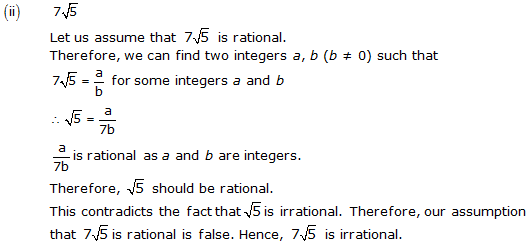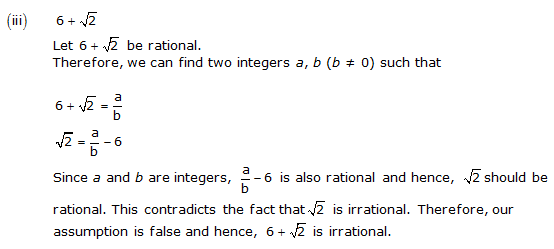# NCERT Solutions for Class 10 Maths Chapter 1 Real Numbers Ex 1.3

NCERT Solutions for Class 10 Maths Chapter 1 Real Numbers Ex 1.3 are part of . Here are we have given Chapter 1 Real Numbers Class 10 NCERT Solutions Ex 1.3.

 Board CBSE Textbook NCERT Class Class 10 Subject Maths Chapter Chapter 1 Chapter Name Real Numbers Exercise Ex 1.3 Number of Questions Solved 3 Category NCERT Solutions

## NCERT Solutions for Class 10 Maths Chapter 1 Real Numbers Ex 1.3

Page No: 14

Question 1
Prove that √5 is irrational.

Solution:
Let us assume, on the contrary, that √5 is a rational number.
Therefore, we can find two integers a,b (b # 0) such that √5 = a\b
Where a and b are co-prime integers.This means that b2 is divisible by 5 and hence, b is divisible by 5.
This implies that a and b have 5 as a common factor.
And this is a contradiction to the fact that a and b are co-prime.
So our assumption that √5 is rational wrong.
Hence,√5 cannot be a rational number. Therefore, √5 is rational.

Concept Insight: There are various ways of proving in mathematics proof by contradiction is one of them. In this approach, we assume something which is contrary to what needs to be proved and arrive at a fact which contradicts something which is true in general. Key result used here is “If P is a prime number and it divides a2then it divides a as well”.

Question 2
Prove that 3 + 2√5 is irrational.

Solution:
Let us assume, on the contrary that 3 + 2√5 is rational,
Therefore, we can find two integers a, b (b ≠ 0) such thatConcept Insight: This problem is solved using proof by contradiction. The key concept used is if p is a prime number then √P is irrational. Do not prove this question by assuming sum of rational and irrational is irrational.

Question 3
Prove that the following are irrationals:
(i) 1/√2
(ii) 7√5
(iii) 6 + √2

Solution:Concept Insight: This problem is solved using proof by contradiction. The key concept used is if p is a prime number then √P is irrational. Do not prove this question by assuming sum or product of rational and irrational is irrational.

We hope the NCERT Solutions for Class 10 Maths Chapter 1 Real Numbers Ex 1.3 help you. If you have any query regarding NCERT Solutions for Class 10 Maths Chapter 1 Real Numbers Ex 1.3, drop a comment below and we will get back to you at the earliest.